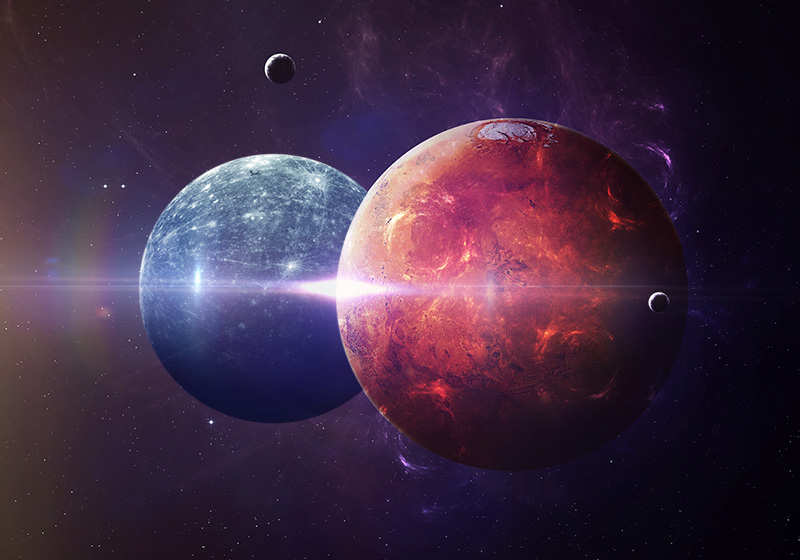## Characteristic Cousins are Found in Two Number Squares

Characteristic Cousins are Found in Two Number Squares. Number squares all have characteristic or featured numbers. The list below describes some of these.  First, consider the 5 x 5.

• It is the sum of numbers 1 -25 = 325.
• Any straight row of five numbers totals 65.
• Any two opposite numbers equals 26.
• The perimeter equals 208. The perimeter is the outside square casing.
• The one central number is 13.

Now consider the larger 8 x 8 in the featured picture:

• The sum of all the numbers 1 – 64 = 2080.
• Any straight row of 8 numbers totals 260.
• Any two opposite numbers = 65.
• The perimeter equals = 910.
• The four central numbers total 130

### Mars and Mercury Are Characteristic Cousins by ancient  use of number SquaresPicture source published on June 28, 2017 Conjunction of Mercury and Mars written by Oranum Psychic Togro

Somehow the 5 x 5 was attributed to Mars. The 8 x 8 called on Mercury. To us today, this seems mysterious.

### Characteristic Cousins All Over the Place

Zero was considered to be a synthetic number in antiquity. Read the internal link below.

## Tens: Here’s the Formula on How Tens Grow into Infinity

With this in mind look at the common characteristic numbers between these two squares. First, I should mention they are both Fibonacci numbers: Five and eight are part of the series that grow by addition of consecutive numbers:  1,1,2, 3, 5, 8,13,21,34… etc. Hence, their number squares also belong to this same  series. These number squares defined key ancient buildings. For this post let’s look some of the common numbers:

•  On the 5 x 5 any straight row of five numbers totals 65. On the 8 x 8 any two opposite numbers = 65.
• On the 5 x 5 any two opposite numbers totals 260. On the 8 x 8 any row totals 260.
• The central number on the 5 x 5 is 13. The perimeter around the 8 x 8 is the sum of the numbers 1 through 13   =91 and then  multiplied by 10.  It duplicates becomes the total of the 910 perimeter around the 8 x 8.

The point is: The designers of Stonehenge and the Great Pyramid both knew and drew on this. More in future posts.